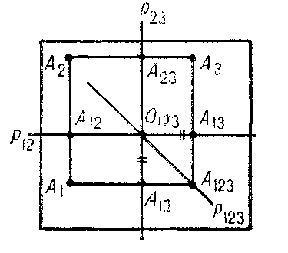# Descriptive geometry

A branch of geometry in which three-dimensional figures, as well as methods for solving and investigating three-dimensional problems, are studied by representing them in the plane. Such representations are constructed by means of central or parallel projection of the figure (nature, an object, an original) on the plane of projection.Figure: d031370a

The most widespread kind of technical drawing is the composite drawing, constructed by means of an orthogonal projection. Essentially, the procedure is as follows. Choose two mutually perpendicular projection planes, \$ \Pi _ {1} \$ and \$ \Pi _ {2} \$. The plane \$ \Pi _ {1} \$ is called the horizontal projection plane, and \$ \Pi _ {2} \$ is the frontal projection plane. Project an arbitrary point \$ A \$ in space orthogonally onto these planes (see Fig. a); this gives the horizontal projection \$ A _ {1} \$ and the frontal projection \$ A _ {2} \$. It is sometimes useful to add a third — the profile projection \$ A _ {3} \$ on the profile plane \$ \Pi _ {3} \$, perpendicular to \$ \Pi _ {1} \$ and \$ \Pi _ {2} \$. To obtain a composite drawing, combining these three projections, make the planes \$ \Pi _ {1} \$ and \$ \Pi _ {3} \$ coincide with \$ \Pi _ {2} \$( the "principal" plane) by rotating them about the lines \$ p _ {12} \$ and \$ p _ {23} \$ in which they intersect \$ \Pi _ {2} \$( see Fig. b). In practice, the position of the projection axes \$ p _ {12} \$ and \$ p _ {13} \$ is usually not marked, i.e. the position of the projection planes is defined only up to a parallel motion.Figure: d031370b

For the construction of more readily visualizable representations, descriptive geometry makes use of an axonometry. To represent objects of considerable extension, one uses drawings obtained by central projection — in other words, in perspective.